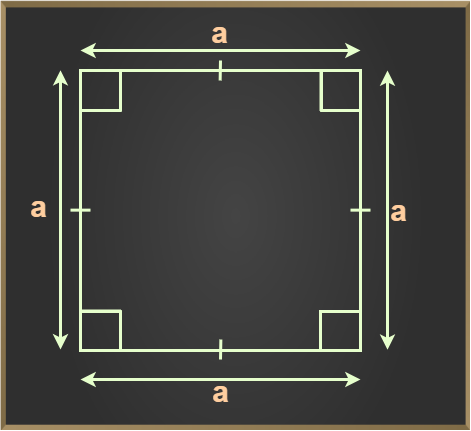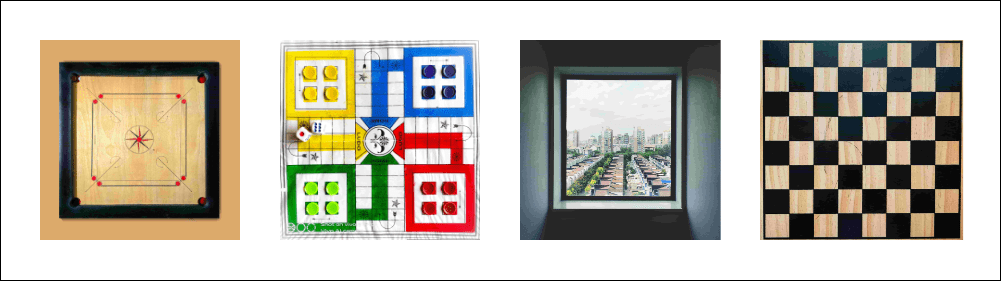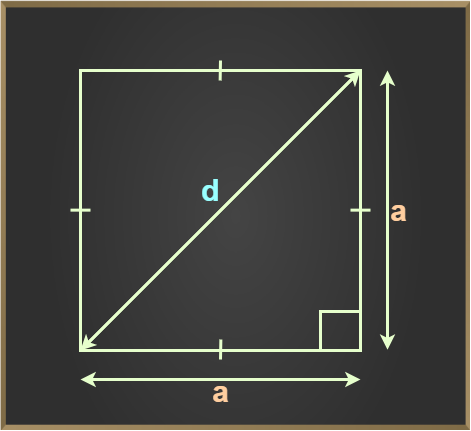GFG App
Open AppBrowser
Continue

A square is a quadrilateral with four equal sides. There are numerous items around us that are square in shape. Each square shape is distinguished by its equal sides and inner angles that are equal to 90°. A square is a closed two-dimensional (2D) form having four sides. It has four equal and parallel sides. A square is a rectangle with equal-length adjacent sides. This indicates that it is a quadrilateral with equal-length sides. Every angle in a square is a right angle. Rhombus also has all four side equal but unlike all its angle are not equal

## What is a Square?

A square is a closed two-dimensional quadrilateral that is made up of two pairs of parallel lines. All four sides of a square are equal and opposite sides of a square are parallel to each other and the interior angles of a square are equal to 90°. The basic figure of a square is shown below.## Definition of Square

A square is defined as a quadrilateral that has:

• All four sides are equal.
• All four angles are equal and the measure of each angle is 90°.
• Opposite pairs of lines are parallel to each other.

## How is a Square Different from a Rhombus?

Square and Rhombus both are Equilateral Quadrilaterals, i.e. both have equal all four sides. The difference between them is that all angles in a square are equal and right angles, but on the other hand all the angles of a rhombus need not be equal.

Hence, Rhombus with right angles is called a Square.

Thus, “Every Square is a Rhombus but all Rhombus are not Squares.”

## Real-Life Examples of Square

A square is a very common shape and can be seen in a variety of objects which we use in our daily lives. Various Square-shaped objects are chess boards, carrom boards, Ludo, etc.## Properties of Square

A square is a closed regular quadrilateral. There are various properties of the square some general properties of the square are given below:

• Square is a quadrilateral with 4 sides and 4 vertices.
• All four sides of the square are equal.
• Opposite pairs of sides of a square are parallel to each other.
• Each interior angle of a square is 90°.
• Diagonals of a square are perpendicular bisectors of each other.
• Diagonals of a square are of equal length.
• Sum of all interior angles of a square is 360°.
• Diagonals of a square divide it into two congruent triangles.

## Formulas of a Square

We know that a square is a four-sided figure with equal sides. There are three basic square formulas that are commonly used in geometry. The first one is to calculate its area, the second is to calculate its perimeter and the third is the diagonal of a square formula. Let us learn these square formulas in detail.

## Area of Square

Area of a square is defined as the total space occupied inside its boundaries. The formula for calculating the area of a square is given as

Area of square = a2

where a is the side of the square

## Perimeter of Square

Perimeter of a square is defined as the length of all its boundaries. Suppose the length of the sides of a square is ‘a’ then its perimeter is given by

Perimeter of Square = Sum of all sides of the square

= a + a + a + a

Perimeter of square = 4a units

As a result, 4a is the perimeter of a square with each side’s length equal to ‘a’ units.

## Diagonal of Square

The diagonals of the square are equal to a√2, where a is the side of the square. The length of both diagonals of a square is equal to each other. The relation between diagonals and sides of a square is given by Pythagoras Theorem.By Pythagoras Theorem, Hypotenuse2 = Base2 + Perpendicular2

Hence,

Diagonal2 = Side2 + Side2

d2 = s2 + s2

d2 = 2s2

d = s√2

where,
d is the length of the diagonal of a square
s is the side of the square.

### Area of Square (when diagonal is given)

If the diagonal of a square is given then the area of the square is given by

Area = d2/2.

where d is the diagonal of the square.

## Solved Examples on Square

Example 1: A square has one of its sides measuring 24 cm. Calculate its area and perimeter.

Solution:

Given, Side of the square = 24 cm

Area of the square: = a2 where, a is the side of the square.

Therefore , Area of the square = a × a

= 24 × 24

= 576 sq cm

Perimeter of the square =  Sum of all sides of square

= a + a + a + a

= 4a

= 4 × 24

= 96 cm

Hence the area of square is 576 sq. cm and the perimeter of square is 96 cm.

Example 2: Find the length of the sides of a square with a perimeter of 56 cm.

Solution:

Given perimeter of the square = 56 cm

Lets suppose length of each side of the square = ‘a’ cm

Therefore, perimeter = 4a cm

56 = 4a

Or a = 56/4

a = 14 cm

Thus, length of each side of square is 14 cm.

Example 3: Find the area of a square whose side is 5m.

Solution:

Given, side (a) = 5 m

Area of the square = a × a

= 5 × 5

= 25 sq m

Example 4: Find the sum of adjacent sides of a square given the perimeter of the square is 16 cm.

Solution:

Given the perimeter of the square = 16 cm

Considering length of each side = ‘a’ cm, the perimeter = 4a cm

Perimeter of Square = Sum of all sides of square

= a + a + a + a

= 4a units

So, 16 = 4a

Or a = 16/4

= 4 cm

Since all sides of a square are of same length, then for the sum of adjacent sides, we can write the sum,

= a + a

= 2a

Therefore, the sum of adjacent sides of the square = 2 × 4 = 8 cm.

Example 5: Find the area of a square park whose perimeter is 420 ft.

Solution:

Given: Perimeter of the square park = 420 ft

As we know that, Perimeter of a square = 4 × side

4 × side = 420

Side = 420/4

Side = 105 ft

Formulae for the Area of a square = side2

Hence, Area of the square park = 1052

= 105 × 105

= 11025 ft2

Thus, the area of a square park whose perimeter is 420 ft is 11025 ft2.

Example 6: A rectangular floor is 40 m long and 30 m wide. Square tiles, each of 4 m side lengths, are to be used to cover the floor. What will be the total number of tiles which will be required to cover the floor?

Solution:

Given, Length of the floor = 40 m, Breadth = 30 m

Area of the rectangular floor = length × breadth = 40 m × 30 m = 1200 sq. m

Side length of one tile = 4 m

Area of one tile = side × side

= 4 m × 4 m

= 16 sq. m

Now number of tiles needed = Total area of floor / area of one tile

= 1200 /16

= 75 tiles

Total 75 tiles are needed to cover the area of rectangular floor of 1200 sq m.

Example 7:  Find the area of a square park whose diagonal is 15 m.

Solution:

Given: Diagonal of the square park = 15 m

Area of a square formula when diagonal is given = d2 / 2

Hence, Area of the square park = 152/ 2

= (15 × 15) / 2

= 225 / 2

= 112.5 sq. m

Thus, the area of a square park whose diagonal is 15 m is 112.5 sq. m.

## FAQs on Square

Question 1: What is a square?

A square is a polygon, with all sides equal and opposite sides parallel to each other. Angles in a square are equal and the measure of each angle is 90 degrees.

Question 2: What is the formula for the area and perimeter of a square?

Area of a square is the region occupied by it. It is equal to the square of its sides.

Area = (side)2

Perimeter of a square is given by adding all its sides.

Perimeter = 4 x side.

Question 3: Can a Rhombus be considered a Square?

No, a rhombus is not a square, but every square can be considered a rhombus.

Question 4: Is a square a polygon?

Square is a closed figure formed by four straight lines So it is considered a quadrilateral. Every quadrilateral is a polygon. So a square is a polygon.

Question 5: Is a square different from a rectangle?